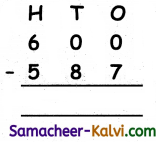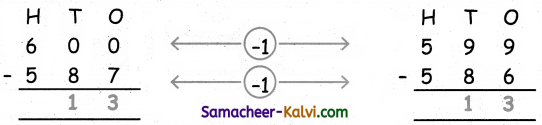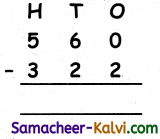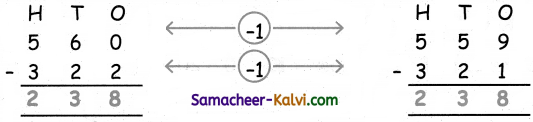## Samacheer Kalvi 3rd Standard Maths Book Solutions Term 3 Chapter 7 Information Processing

Quick ways of adding (Text Book Page No. 49):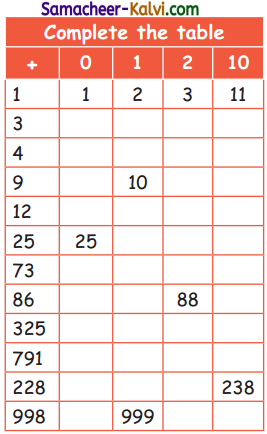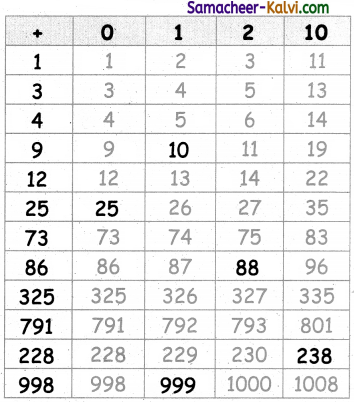Exercise (Text Book Page No. 50):

Find the sum of

i) 5 + 1 + 5 + 9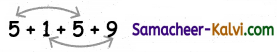5 + 5 = 10
9 + 1 = 10
Hence the sum = 10 + 10 = 20

ii) 2 + 5 + 5 + 7 + 1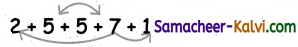2 + 7 + 1 = 10
5 + 5 = 10
Hence the sum = 10 + 10 = 20

iii) 3 + 6 + 1 + 2 + 8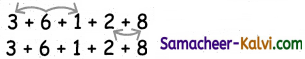3 + 6 + 1 = 10
8 + 2 = 10
Hence the sum = 10 + 10 = 20Add the given big numbers using the above method (Text Book Page No. 51):

Question 1.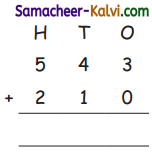543 = 500 + 40 + 3
210 = 200 + 10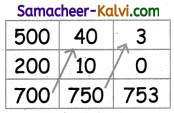Question 2.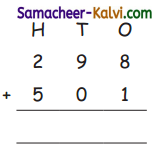298 = 200 + 90 + 8
501 = 500 + 1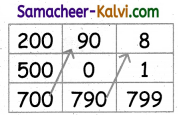Question 3.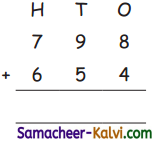798 = 700 + 90 + 8
654 = 600 + 50 + 4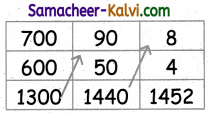Question 4.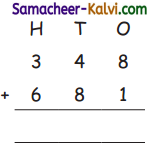348 = 300 + 40 + 8
681 = 600 + 80 + 1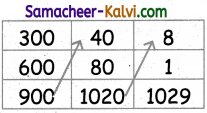Question 5.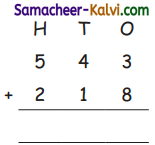543 = 500 + 40 + 3
218 = 200 + 10 + 8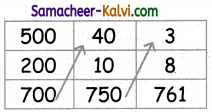Question 6.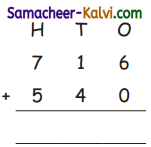716 = 700 + 10 + 6
540 = 500 + 40 + 0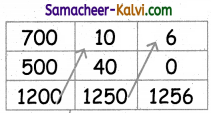Quick ways of subtracting (Text Book Page No. 52):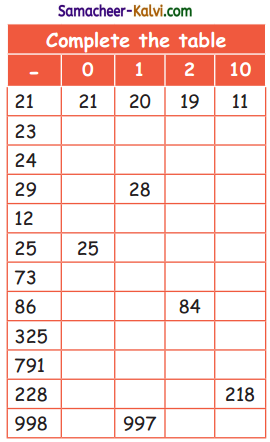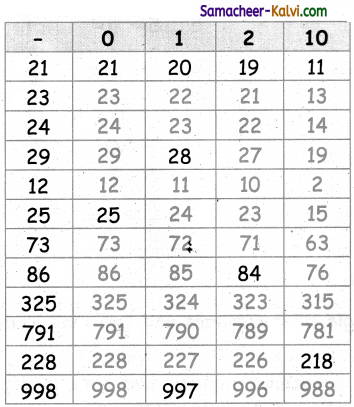Subtract the given numbers using the above method (Text Book Page No. 53):

Question 1.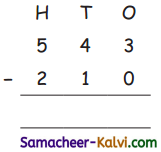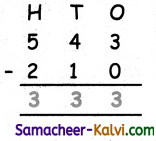Question 2.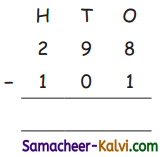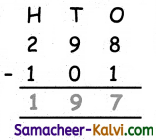Question 3.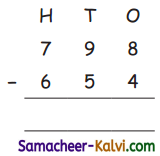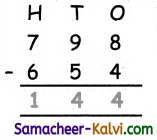Question 4.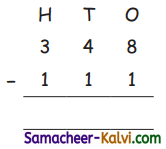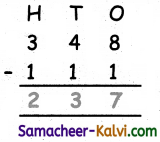Question 5.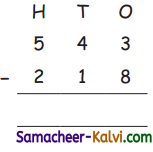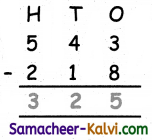Question 6.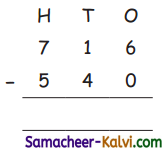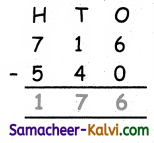Question 7.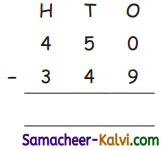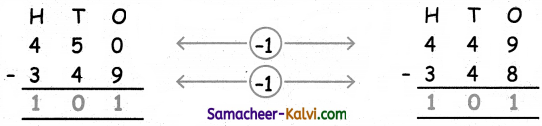Question 8.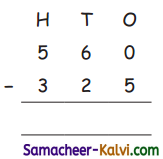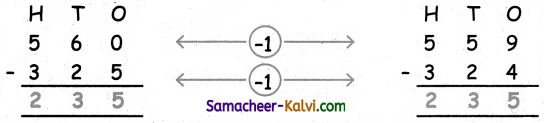Question 9.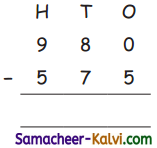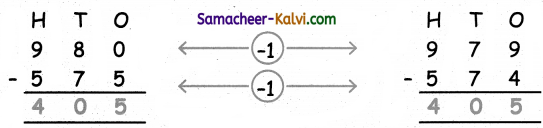Mental Arithmetic (Text Book Page No. 54):

Able to add and subtract single digit numbers and two digit numbers mentally:

Question 1.
In a tree planting ceremony, 6 coconut saplings and 5 neem saplings are planted. Find the total number of saplings planted.
6 + 5 = 11

Question 2.
There are 5 tender coconuts in a tree. If they pick 3 tender coconuts, then find the remaining tender coconuts?
5 – 3 = 2

Question 3.
46 boys and 27 girls visited a park on Sunday. Find the total number of boys and girls who visited the park?
46 + 27 = 73Question 4.
50 pens are in a box. Out of which, 34 pens are given to the students. Find the number of pens left?
50 – 34 = 16

Question 5.
70 balls are given to 7 persons. How many balls are given to each person?
70 ÷ 7 = 10 [10 × 7 = 70]

Question 6.
8 books can be arranged in a row. In how many rows can 48 books be arranged?
48 ÷ 8 = 6 [8 × 6 = 48]

Question 7.
10 pens can be kept in a box. How many boxes are needed to keep 100 pens?
100 ÷ 10 = 10Question 1.
Find the sum of

(i) 7 + 7 + 3 + 3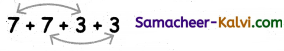7 + 3 = 10 and
7 + 3 = 10
Hence the sum of the number = 10 + 10 = 20

(ii) 6 + 4 + 4 + 6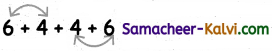6 + 4 = 10 and
4 + 6 = 10
Hence the sum of the number = 10 + 10 = 20Question 2.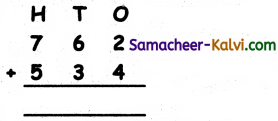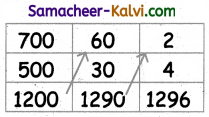Question 3.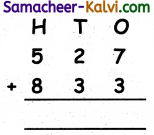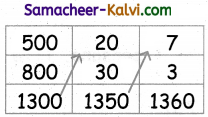Question 4.
Subtract